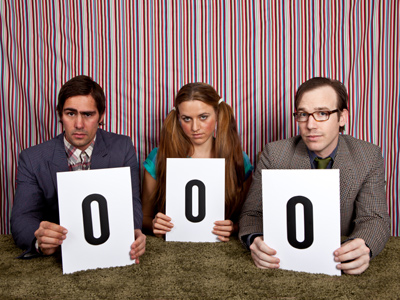0 lies between -1 and 1 on a numberline.

# Compare and Order Numbers (Year 4)

One of the most important skills children develop in KS2 Maths is the ability to compare and order numbers. This includes ordering negative numbers, decimal numbers and four figure numbers, as well as recognising the greater than >, smaller than < and equal to = symbols. This is the first of two quizzes on the subject and it is aimed primarily at children in Year 4.

Being able to compare and order numbers is about knowing the size of numbers. Knowing 1 to 100 is straightforward, but what about negative numbers or numbers with decimal points? Is 0.7 larger than 0.07? What about larger numbers like 9,973 and 9,987 - which is larger? If you know your ones tens, hundreds and thousands, along with your tenths, hundredths and thousandths, you should be able to work the answers out.

Try this quiz and see how well you can compare and order whole, decimal and negative numbers. For 8-9 year olds.

1.
Which is the longest distance?
9,412 m
9,214 m
9,421 m
9,241 m
9,421 and 9,412 both have 9 thousands and 4 hundreds but 9,421 has 2 tens while 9,412 only has 1
2.
Which number is fewer than 53?
45
54
68
55
I hope you got this one right!
3.
Which is the smallest number?
5,478
5,748
5,847
5,784
All of the numbers have 5 thousands but 5,478 only has 4 hundreds, while all the rest have 7 or 8
4.
What does this symbol mean >?
Less than
Greater than
Equal to
Greater than or equal to
The largest part of the symbol will be next to the largest number e.g. 3 > 2
5.
What does this symbol mean < ?
Less than
Greater than
Equal to
Greater than or equal to
The smallest part of the symbol will be next to the smallest number e.g. 2 < 3
6.
Which of these numbers is greater?
4,561
4,516
4,615
4,651
4,651 and 4,615 both have 4 thousands and 6 hundreds but 4,651 has 5 tens while 4,615 only has 1
7.
Which of these numbers is the largest?
0.2
1.3
0.9
1.1
Decimals can be confusing. If two numbers share the same ones (like 1.3 and 1.1) then the one with the highest tenths is higher
8.
Which number comes next? -3, -2, -1
0
1
2
3
0 is between -1 and 1
9.
Which number comes after -4 on a number line, counting down?
-2
-3
-5
-1
A number line with negative numbers would be -5, -4, -3, -2, -1
10.
Which number lies halfway between 1,020 and 1,050?
1,030
1,035
1,040
1,045
1,020 + 15 = 1,035 and 1,050 - 15 = 1,035 too
You can find more about this topic by visiting BBC Bitesize - Compare numbers up to 1000

Author:  Amanda Swift